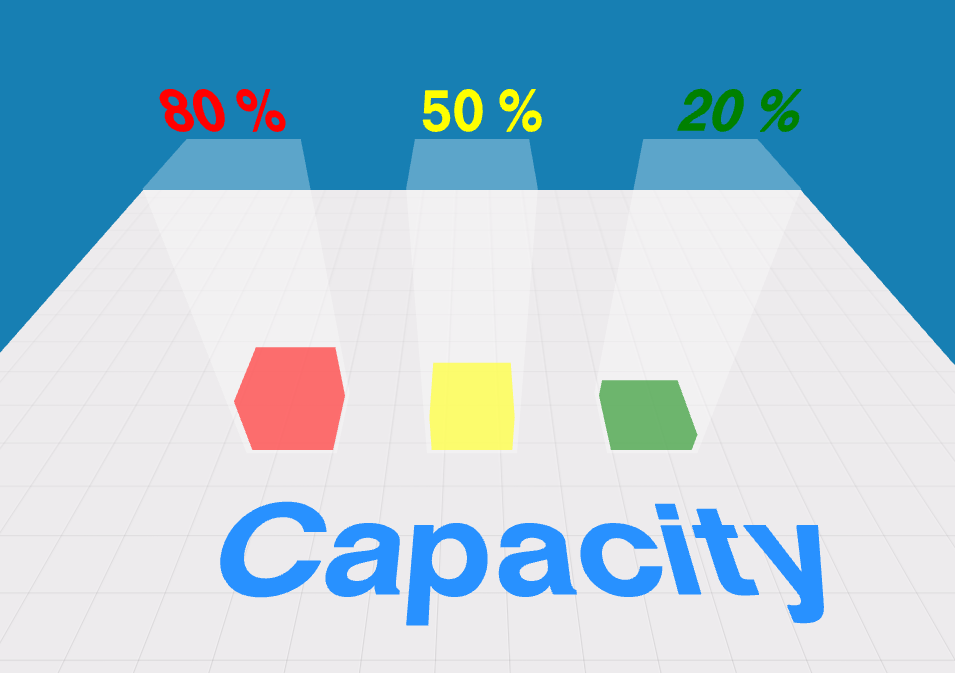# Threejs教程——动画

```    var render = function () {
requestAnimationFrame(render);
step += 0.01;
cube.position.x = 2 + (1 * (Math.cos(step)));
cube.position.y = 2 + (10 * Math.abs(Math.sin(step)));
cube.rotation.x += 0.03;
cube.rotation.y += 0.03;
cube.rotation.z += 0.03;
renderer.render(scene, camera);
};
```

```<script src="js/Tween.js"></script>
```

```var tweenPosition = new TWEEN.Tween(cubeMesh.position)
.to({ x: cubeMesh.position.x, y: height * 0.5 * full, z: cubeMesh.position.z }, 1500)
.easing(TWEEN.Easing.Back.Out)
.start();
```

```var render = function () {
requestAnimationFrame(render);
TWEEN.update();
renderer.render(scene, camera);
};
```

```       var tweenScale = new TWEEN.Tween(cubeMesh.scale)
.to({ x: 1, y: height * full, z: 1 }, 1500)
.easing(TWEEN.Easing.Back.Out)
.start();
var tweenPosition = new TWEEN.Tween(cubeMesh.position)
.to({ x: cubeMesh.position.x, y: height * 0.5 * full, z: cubeMesh.position.z }, 1500)
.easing(TWEEN.Easing.Back.Out)
.start();
```2016年12月29日 1593 浏览 评论